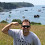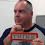## Wednesday, September 20, 2017

### REGEXP for searching in stringsContinuing my posts about the regular expressions introduced as part of IBM i Technical Refreshes to 7.2, TR6, and 7.3, TR2, I have struggled to come up with a good brief description that would suffice for this post's title. IBM's documentation describes this function as:

returns the starting position or the position after the end of the matched substring

After playing with this function for awhile I would describe it as: "returns the position where the searched pattern occurs, whether first or nth occurrence, in the source string". And I would have to admit that description is better than IBM's, but it not a good description either. It is probably best I start explaining how to use this function and you will be able to decide how you would describe it.

The format for this function is as follows:

 ``` REGEXP_INSTR(source-string, search-pattern, starting-position, occurrence,position-value, flags, group) ```

Some of the parameters' function is obvious, others less so.

1. source-string  The variable or string, that will be searched.
2. search-pattern  What I am looking for in the source string.
3. starting-position  Where I want to start searching in my source string.
4. occurrence  Do I want the first, second, third, etc. occurrence of the search pattern in the source string.
5. position-value
• Zero ( 0 ), which is the default, return to me the starting position of the search pattern.
• 1 return to me the ending position of the search pattern.
6. flags  These are the same flags used for the REGEXP_LIKE.
7. group  Capture group, I have to admit I did not find a reason why I would use this.

I am going to give examples of using this function in two ways:

1. Using a Select statement, I would use this if I was using an SQL client, such as STRSQL.
2. Using a Set statement, as I would if I was testing a string in a program.

Let's get started. Here is a Select statement containing the most basic form of the expression:

 ```SELECT REGEXP_INSTR('RPGPGM.COM is the best website for rpg examples', 'o',1,1) FROM SYSIBM.SYSDUMMY1 ```

In this statement I am searching the string for the lower case letter o only, starting in the first position, and for the first occurrence of a lower case o. As this is a Select statement it needs to be performed using a table/file, in this case I am using IBM's dummy table, SYSDUMMY1 in the library SYSIBM. The statement returns the following value:

 ```REGEXP_INSTR 33 ```

What if I wanted to return the position of the first letter o of either case? I can use the lower case i in the flags perimeter, and I have to give a value in the position value too.

 ```SELECT REGEXP_INSTR('RPGPGM.COM is the best website for rpg examples', 'o',1,1,0,'i') FROM SYSIBM.SYSDUMMY1 ```

The position value, fifth parameter, is 0 (zero) as I want to return where the search pattern starts in the string.

This returns:

 ```REGEXP_INSTR 9 ```

What happens when I change the position value to 1?

 ```SELECT REGEXP_INSTR('RPGPGM.COM is the best website for rpg examples', 'o',1,2,1,'i') FROM SYSIBM.SYSDUMMY1 ```

The value 10 is returned as that is where the current search pattern ends in the string.

 ```REGEXP_INSTR 10 ```

And what about finding, for example, the second occurrence of the letter e in the search string?

I change the fourth parameter, occurrence, to 2.

 ```SELECT REGEXP_INSTR('RPGPGM.COM is the best website for rpg examples', 'e',1,2,0,'i') FROM SYSIBM.SYSDUMMY1 ```

I am returned 20, which, of course, is where in the search string the second e is found.

 ```REGEXP_INSTR 20 ```

A better example of using the occurrence parameter is shown in the RPG example code below.

I create this program to show how I would do all of what I described above in a RPG program. Let me start with the definitions of the variables I will be using.

 ```01 **free 02 dcl-s String char(47) inz('RPGPGM.COM is the best website for rpg examples') ; // ----+----1----+----2----+----3----+----4----+-- 03 dcl-s Position int(5) ; 04 dcl-s Counter like(Position) ; ```

Line 1: This program is in totally free RPG, as it is 2017 why would I be writing in anything else?

Line 2: This is the definition of the variable to contain the search string I will be using. I created a ruler in a comment line below to make it easier to see where the various characters are.

Line 3: I will be using Position to contain the value returned by the SQL statements.

Line 4: Counter will be used to condition a For-loop.

Now onto the first example: returning the first occurrence of (lower case) o. My personal preference is to use SET, rather than SELECT, if I am not getting data from a table/file.

 ```05 exec sql SET :Position = REGEXP_INSTR(:String,'o',1,1) ; 06 dsply ('1 = ' + %char(Position)) ; ```

The RPG variables used in the SQL statement need to be prefixed with :, so that the compiler knows this is a program variable.

When the program is run I see the following displayed:

 ``` DSPLY 1 = 33 ```

In the next example I wanted to find any letter o, regardless of case.

 ```07 exec sql SET :Position = REGEXP_INSTR(:String,'o',1,1,0,'i') ; 08 dsply ('2 = ' + %char(Position)) ; ```

And I find the first one in the ninth position of the search string.

 ``` DSPLY 2 = 9 ```

In RPG I can illustrate better how to use the occurrence parameter to find multiple occurrences of a letter in the search string.

 ```09 for Counter = 1 by 1 to 47 ; 10 exec sql SET :Position = REGEXP_INSTR(:String,'e',1,:Counter,0,'i') ; 11 dsply ('3 = ' + %char(Counter) + ':' + %char(Position)) ; 12 if (Position = 0) ; 13 leave ; 14 endif ; 15 endfor ; ```

Line 9: I am using the variable Counter as the counter for the For-loop. I am looping up to 47 as that is the length of the search string variable.

Line 10: The first time through Counter is 1, so it finds the first occurrence of e. Second time through I am looking for the second occurrence, etc..

Line 11: I am using the Display operation code to display the value of Counter followed by the position the e is found in.

Lines 12 – 14: If an occurrence of e cannot be found, then zero is returned from the SQL statement. As there are no occurrences to look for it is time to quit the For-loop.

When run I run this code I see:

 ``` DSPLY 3 = 1:17 DSPLY 3 = 2:20 DSPLY 3 = 3:25 DSPLY 3 = 4:30 DSPLY 3 = 5:40 DSPLY 3 = 6:46 DSPLY 3 = 7:0 ```

The seventh loop returned zero as there are only six e in the search string.

If you are interested in learning what else you can use the REGEXP_INSTR for visit IBM's KnowledgeCenter page, linked below, and use your favorite search engine to search for REGEXP_INSTR as much of what Oracle's version can do I have found the IBM i will do the same.

Other Db2 for i regular expressions:

This article was written for IBM i 7.3 TR2 and 7.2 TR6 only.

1.Great info I can see how this might help with certain parsing routines.
*FYI line-9 2nd to last word is STRONG seems like you meant STRING

2.Thank you for bringing the spelling error to my attention. It has now been fixed.

3.Power i and modern powerful pattern searching with regular expressions. Know the tools you have so you will know when to use them and how.

4.Nice article. Thanks.

Just a note.
Instead of using SYSDUMMY1, we can use the command VALUES.
i.e: VALUES(REGEXP_INSTR('RPGPGM.COM is the best website for rpg examples','o',1,2,1,'i'));

5.You always have helpful posts, whether as review or learning something new. I appreciate it. It's nice to see a very powerful Unix function in SQL. One correction to the above:

"The position value, fourth parameter, is 0 (zero)". I think you meant the fifth parameter.

1.Thank you for bringing that error to my attention. It has now been fixed.

6.You mentioned that you are changing the position value from 0 to 1. However, you also changed the occurrence from 1 to 2 in the below query. Hence, the result is 34 and not 10 as intended

SELECT
REGEXP_INSTR('RPGPGM.COM is the best website for rpg examples',
'o',1,2,1,'i')
FROM SYSIBM.SYSDUMMY1

To prevent "comment spam" all comments are moderated.
```